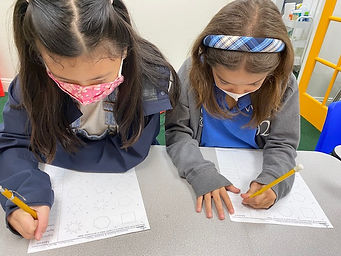## Ms. Dawn

### Target 1​

###### Lesson Type:

Continuation

Geometry

:

Shape

Classify 2D and 3D figures based on the basic properties of shapes.

###### 1:

Identify quadrilaterals by their defining attributes (number of sides, number of vertices, vertices angle measurements, line types, and side lengths).

###### 2:

Identify triangles by their defining attributes (number of sides, number of vertices, vertices angle measurements, and side lengths).

###### 3:

Identify parallel and perpendicular lines.

###### 4:

Differentiate between regular and irregular polygons.

###### 5:

Differentiate between convex and concave polygons.

4th

###### Vocabulary:

Base, Faces, Edges, Vertices, Sides, Parallel lines, Perpendicular lines, Intersecting lines, Regular polygons, Irregular polygons, Convex, Concave

Activities:

1. Reviewed the attributes of how 3D shapes are defined. Discussed how the base of the shape defines its name. After reviewing that idea, students identified a variety of 3D shapes by all the different attributes.

2. After identifying the shapes, they identifed that amount of faces, edges, and vertices.

3. Students were introduced to the vocabulary terms perpendicular, intersecting and parallel lines. Students drew a few examples of each on their whiteboard. Talked about the attributes that identify intersecting, parallel and perpendicular lines.

4. Students answered a variety of questions relating to a figure that showed parallel, perpendicular and intersecting lines.

5. Next, they used a road map to identify and draw roads relating to parallel, perpendicular, and intersecting lines.

6. Discussed the defintion of regular and irregular shapes. Students drew a variety of shapes to show their understanding of the vocabulary terms.

7. Students then were given a variety of shapes and needed to identify which ones are irregular and which ones were regular. Also, address why that was the case.

8. Finally discussed the terms concave and convex. Students drew a variety of shapes to show their thinking for each word.

9. Lastly, students sorted shapes in the correct category, either concave or convex. Also, identified which of those shapes were not polygons.### Home Exploration

###### Guiding Questions:## Absent Students:

### Target 2

:

###### Vocabulary:

Activities:### Home Exploration

###### Guiding Questions:### Target 3

:

###### Vocabulary:

Activities:### Home Exploration